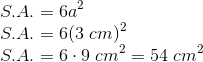# Intermediate Geometry : How to find the surface area of a cube

## Example Questions

### Example Question #21 : Cubes

One side of a cube is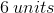long. What is the surface area of the cube?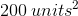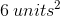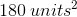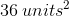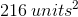Explanation:

To find the surface area of a cube, we find the area of a face by multiplying two of its sides together; then, we multiply by, since a cube has six faces. So, ifis the length of one side of a cube, then the cube's surface area can be represented as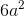.

We know that for this problem,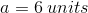, so we can substitute this value into the equation and solve for the cube's surface area: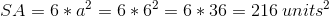### Example Question #31 : Cubes

The volume of a cube is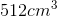.

What is the surface area of the cube?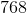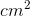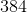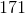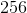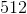Explanation:

Using the volume given, we take it's cube to find the length of the cube: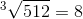cm.

Therefore, the length of the cube is 8 cm.

Knowing the properties of a cube, this implies that the width and height of the cube is also 8 cm.

Since all sides are identical, the formula for the surface area is length times width times the number of sides: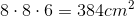.

### Example Question #32 : Cubes

If a side of a cube has a length of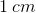, what is the cube's surface area?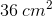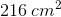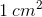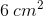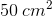Explanation:

Write the formula to find the surface of a cube, whereis the length.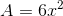Substitute and solve.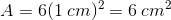### Example Question #1 : How To Find The Surface Area Of A Cube

Find the surface area of a cube with a side length of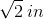.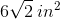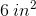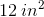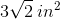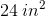Explanation:

Write the formula for the surface area of a cube, substitute the length provided in the question, and simplify.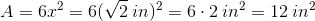### Example Question #34 : Cubes

if the side length of a cube is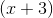, what is the cube's surface area?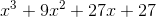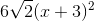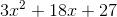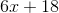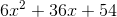Explanation:

The formula for the surface area of a cube is: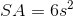, whereis the length of one side of the cube.

We are given the length of one side of the cube in question, so we can substitute that value into the surface area equation and solve: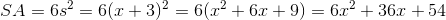### Example Question #35 : Cubes

A cube has a sphere inscribed inside it  with a diameter of 4 meters. What is the surface area of the cube?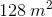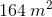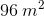None of these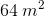Explanation:

Since the sphere is inscribed within the cube, its diameter is the same length as an edge of the cube. Since cubes have identical side lengths we find the area of one side and then multiply by the number of sides to find the total surface area.

Area of one side: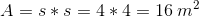Total surface area: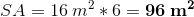### Example Question #36 : Cubes

A geometric cube has a volume of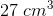. Find the surface area of the cube.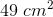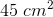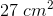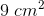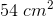Explanation:

We first need to know the edge length before we can solve for surface area. Since we are provided the volume and all edges are of equal length, we can use the formula for volume to get the length of sides: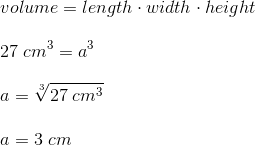Now that we know the length of sides, we can plug this value into our surface area formula: